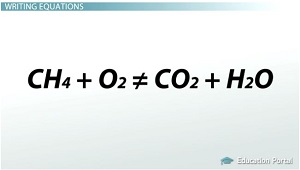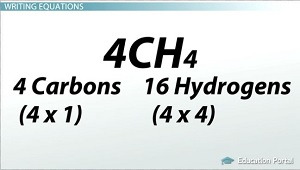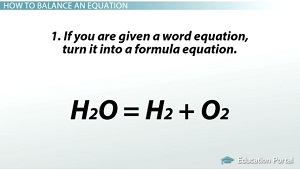# Chemical Reactions and Balancing Chemical Equations

An error occurred trying to load this video.

Try refreshing the page, or contact customer support.

Coming up next: Mole-to-Mole Ratios and Calculations of a Chemical Equation

### You're on a roll. Keep up the good work!

Replay
Your next lesson will play in 10 seconds
• 0:07 Conservation of Matter
• 0:36 Writing Equations
• 1:55 How to Write a…
• 4:16 Practice Equations
• 5:29 Lesson Summary

Want to watch this again later?

Timeline
Autoplay
Autoplay
Speed

#### Recommended Lessons and Courses for You

Lesson Transcript
Instructor: Amy Meyers

Amy holds a Master of Science. She has taught science at the high school and college levels.

In this lesson, you'll learn how to balance a chemical reaction equation using the conservation of matter law. You'll also learn how to write both word and formula equations, what the subscripts after a letter mean and what the numbers in front of compounds mean.

## Conservation of Matter

Conservation of matter is a basic law of physics that was discovered in the 19th century. Essentially what it means is that matter is never created or destroyed. It can change into energy, but it never disappears. Although the law is considered to be a law of physics, it also applies to chemistry. In a chemical equation, the law of conservation of matter must be met. This means that whatever amount of reactants you started with, this is the amount of product you end up with.## Writing Equations

Remember that equations can be written in word form, such as methane + oxygen = carbon dioxide + water, but more often they are written in a shorthand fashion called a formula equation. This looks like CH4 + O2 = CO2 + H2 O. There is something wrong with this equation, though. This equation is not a balanced equation. To be correctly written, it must have the same amount of elements on both sides of the equation, and if you look, it doesn't. It has four hydrogens on the left, but only two hydrogens on the right. That means matter was destroyed somewhere, and we know that can't happen.

When writing your formula equations, remember that a subscript refers to the atom directly before it. So, in CH4, the 4 means four hydrogen atoms. Also, remember if there is a number in front of a compound, that number applies to all the atoms in that compound. The number in front of a compound or element is called the coefficient. When there isn't a number there, it is assumed to be the number 1. So, if I put a 4 in front of CH4, so it looked like 4CH4, that would mean I have four carbons (four times one) and 16 hydrogens (four times four).## How to Balance an Equation

To write a balanced equation, here are the steps:

Step 1: If you are given a word equation such as water = hydrogen + oxygen, turn it into a formula equation. H2 O = H2 + O2.

Step 2: Balance the different types of atoms one at a time.

Step 2a: First balance the atoms that are combined and appear only once on each side of the equation.

Step 2b: Balance other compounds that appear on both sides of the equation.

Step 2c: Balance the hydrogen (H) atoms and oxygen (O) atoms last.

Step 3: Count the atoms on each side of the equation to make sure it is balanced.

So, here is the way it would work: You are given water = hydrogen + oxygen.

Step 1: You rewrite it as H2 O = H2 + O2.

Step 2 a: Balance the atoms that are combined and only appear once. Hmmm. This doesn't apply too much in this example.Step 2b: There are no compounds on either side, so skip this step.

To unlock this lesson you must be a Study.com Member.

### Register to view this lesson

Are you a student or a teacher?

### Unlock Your Education

#### See for yourself why 30 million people use Study.com

##### Become a Study.com member and start learning now.
Back
What teachers are saying about Study.com

### Earning College Credit

Did you know… We have over 160 college courses that prepare you to earn credit by exam that is accepted by over 1,500 colleges and universities. You can test out of the first two years of college and save thousands off your degree. Anyone can earn credit-by-exam regardless of age or education level.